# Selina Concise Mathematics Class 6 ICSE Solutions Chapter 26 Triangles

## Selina Concise Mathematics Class 6 ICSE Solutions Chapter 26 Triangles (Including Types, Properties and Constructions)

Selina Publishers Concise Mathematics Class 6 ICSE Solutions Chapter 26 Triangles (Including Types, Properties and Constructions)

### Triangles Exercise 26A – Selina Concise Mathematics Class 6 ICSE Solutions

Question 1.
In each of the following, find the marked unknown angles :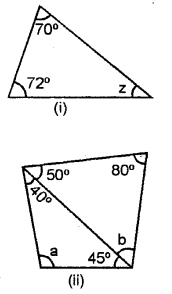Solution: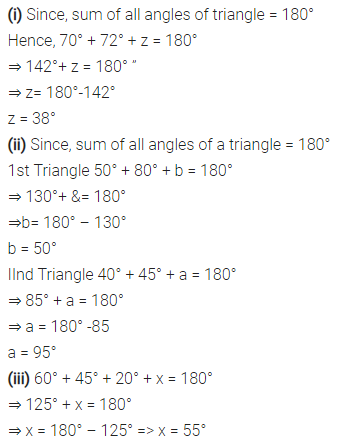Question 2.
Can a triangle together have the following angles ?
(i) 55°, 55° and 80°
(ii) 33°, 74° and 73°
(iii) 85°, 95° and 22°.
Solution:Question 3.
Find x, if the angles of a triangle are:
(i) x°, x°, x°
(ii) x°, 2x°, 2x°
(iii) 2x°, 4x°, 6x°
Solution:Question 4.
One angle of a right-angled triangle is 70°. Find the other acute angle.
Solution:Question 5.
In ∆ABC, ∠A = ∠B = 62° ; find ∠C.
Solution: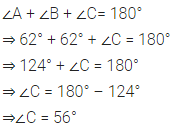Question 6.
In ∆ABC, C = 56°C = 56° ∠B = ∠C and ∠A = 100° ; find ∠B.
Solution: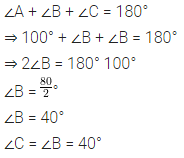Question 7.
Find, giving reasons, the unknown marked angles, in each triangle drawn below: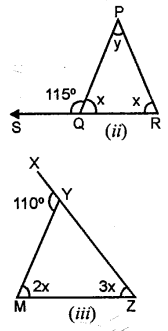Solution:Question 8.
Classify the following triangles according to angle :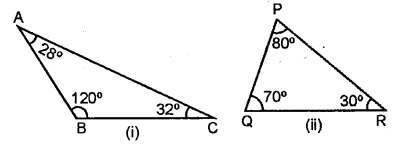Solution:Question 9.
Classify the following triangles according to sides :Solution:### Triangles Exercise 26B – Selina Concise Mathematics Class 6 ICSE Solutions

Construct traingle ABC, when :
Question 1.
AB = 6 cm, BC = 8 cm and AC = 4 cm.
Solution:Question 2.
AB = 3.5 cm, AC = 4.8 cm and BC = 5.2 cm.
Solution: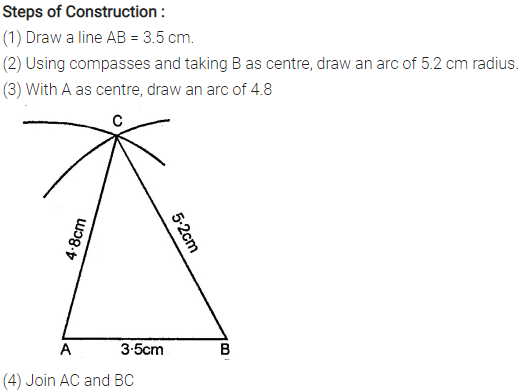Question 3.
AB = BC = 5 cm and AC = 3 cm. Mea¬sure angles A and C. Is ∠A = ∠C?
Solution: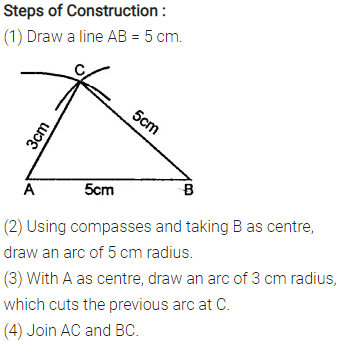Question 4.
AB = BC = CA = 4.5 cm. Measure all the angles of the triangle. Are they equal ?
Solution:Question 5.
AB = 3 cm, BC = 7 cm and ∠B = 90°.
Solution:Question 6.
AC = 4.5 cm, BC = 6 cm and ∠C = 60°.
Solution:Question 7.
AC = 6 cm, ∠A = 60“ and ∠C = 45°. Measure AB and BC.
Solution:Question 8.
AB = 5.4 cm, ∠A = 30° and ∠B = 90°. Measure ∠C and side BC.
Solution:Question 9.
AB = 7 cm, ∠B = 120° and ∠A = 30°. Measure AC and BC.
Solution:Question 10.
BC = 3 cm, AC = 4 cm and AB = 5 cm. Measure angle ACB. Give a special name to this triangle.
Solution:### Triangles Revision Exercise – Selina Concise Mathematics Class 6 ICSE Solutions

Question 1.
If each of the two equal angles of an isosceles triangle is 68°, find the third angle.
Solution:Question 2.
One of the angles of a triangle is 110°, the two other angles are equal. Find their value.
Solution:Question 3.
The angles of a triangle are in the ratio 3:5: 7. Find each angle.
Solution:Question 4.
The angles of a triangle are (2x – 30°),(3x – 40°) and ($$\frac { 5 }{ 2 }$$x + 10°) Find the value of x .
Solution: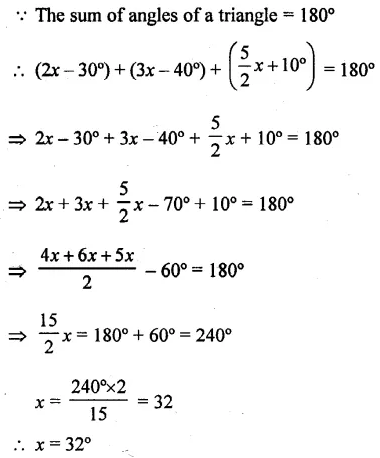Question 5.
In each of the following figures, triangle ABC is equilateral and triangle PBC is isosceles. If PBA = 20°; find in each case:
(a) angle PBC.
(b) angle BPC.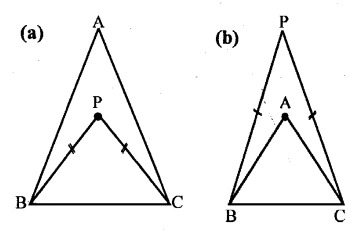Solution:Question 6.
Construct a triangle ABC given AB = 6 cm, BC = 5 cm and CA = 5.6 cm. From vertex A draw a perpendicular on to side BC. Measure the length of this perpendicular.
Solution:Question 7.
Construct a triangle PQR, given PQ = 6 cm, ∠P = 60° and ∠Q = 30°. Measure angle R and the length of PR.
Solution:Question 8.
Construct a triangle ABC given BC = 5 cm, AC = 6 cm and ∠C = 75°. Draw the bisector of the interior angle at A. Let this bisector meet BC at P ; measure BP.
Solution:Question 9.
Using ruler and a pair compass only, construct a triangle XYZ given YZ = 7 cm, ∠XYZ = 60° and ∠XZY = 45°. Draw the bisectors of angles X and Y.
Solution:Question 10.
Using ruler and a pair compass only, construct a triangle PQR, given PQ = 5.5 cm, QR = 7.5 cm and RP = 6 cm. Draw the bisectors of the interior angles at P, Q and R. Do these bisectors meet at the same point ?
Solution: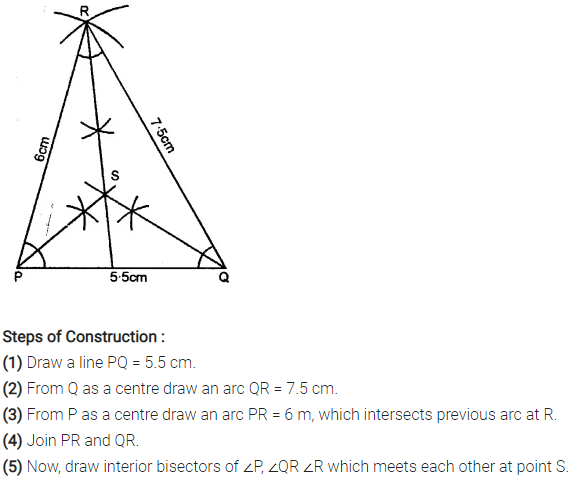Question 11.
One angle of a triangle is 80° and the other two are in the ratio 3 : 2. Find the unknown angles of the triangle.
Solution:Question 12.
Find the value of x if ∠A = 32°, ∠B = 55° and obtuse angle AED = 115°.
Solution: